• ## 傅里叶级数与傅里叶变换

万次阅读 多人点赞 2019-07-19 17:40:05
傅里叶级数：任何周期函数，只要满足一定条件都可以表示为不同频率的...傅里叶级数与傅里叶变换的关系：周期函数的周期可以趋向无穷大，这样就可以将傅里叶变换看成是傅里叶级数的推广。 一、傅里叶级数 1....
傅里叶级数：任何周期函数，只要满足一定条件都可以表示为不同频率的正弦和/或余弦之和的形式，该和成为傅里叶级数。

傅里叶变换：任何非周期函数（但该曲线下的面积是有限的），也可以用正弦和/或余弦乘以加权函数的积分来表示，在这种情况下的公式就是傅里叶变换。

傅里叶级数与傅里叶变换的关系：周期函数的周期可以趋向无穷大，这样就可以将傅里叶变换看成是傅里叶级数的推广。

一、傅里叶级数

1.1傅里叶级数的三角形式

假设f(x)是周期为T的函数，并且满足傅里叶级数的收敛条件，那么可以写作傅里叶级数：

（1）

其中：

（2）

（3）

（4）

1.2傅里叶级数的复指数形式

借助欧拉公式可以将上述的傅里叶级数的三角形式转换为如下的复指数形式：

(5)

其中：

(6)

也就是说，曲线可以理解为无数旋转的叠加，将f(x)看作是圆周运动的组合，只是x不断增大，不是绕着圆变换。X的不断增大，称为时域。

1.3傅里叶级数的余弦形式（略）

二、傅里叶变换

2.1一维连续傅里叶变换

对于定义域为整个时间轴（-∞<t<+∞）的非周期函数f（x），此时已无法通过周期拓延将其扩展为周期函数，这种情况下就要用到傅里叶变换：

(7)

根据傅里叶反变换得出：

(8)

其中u=1/T,是函数f(x)的频率。

仔细观察式（7）和式（8），对比复指数形式的傅里叶级数展开式（5），注意到在这里傅里叶变换的结果F（u）实际上相当于傅里叶级数展开中的傅里叶系数，而反变换公式（8）则体现出不同频率复指数函数的加权和的形式，相当于复指数形式的傅里叶级数展开公式，只不过这里的频率u变为了连续的，所以加权和采用了积分的形式。这是因为随着作为式（6）的积分上下限的T向整个实数定义域的扩展，即T趋近于∞，频率u则趋近于du（u=1/T），导致原来离散变化的u连续化。

2.2一维离散傅里叶变换

一维函数f（x）（其中x=0,1,2,...,M-1）的傅里叶变换的离散形式为：

(9)

相应的反变换为：

(10)

仔细观察式（9）和式（10）注意到在频率域下变换F（u）也是离散的，且其定义域仍为0-M-1,这是因为F（u）的周期性，即：

式（10）中的系数1/M，在这里被放在了反变换之前，实际上它也可以位于式（9）的正变换中。更一般的情况是只要能保证正变换和反变换之前的系数乘积为1/M即可。例如：两个公式的系数可以均为。

为了求得每一个F（u）（u=0,1,2,...,M-1），需要全部M个点的f（x）都参与加权求和运算。对于M个u，则总共需要大约M2次计算。当图像较大时，计算量较大。在快速傅里叶变换中会研究如何提高速度。

2.3二维连续傅里叶变换

（11）

其反变换为：

（12）

2.4二维离散傅里叶变换

在数字图像处理领域，我们关注的是二维离散函数的傅里叶变换（Discrete Fourier Transform,DFT）公式：

(13)

其反变换为：

(14)

其中M、N分别为图像的宽度、高度。

相对于空间域的变量x,y，这里的u，v是频率域的变量。同上述一维中的情况相同，由于频谱的周期性，式（13）只需对u值（u=0,1,2,...,M-1）及v值（v=0,1,2,...,M-1）进行计算。系数1/MN的位置并不重要。

根据式（13），频域原点位置的傅里叶变换为：

(15)

显然这是f(x,y)各个像素的灰度之和。而如果将系数1/MN放在正变换之前，则F（0,0）对应于原图像f（x，y）的平均灰度。F(0,0)有时被称作频率谱的直流分量。

三、频谱和功率谱

3.1频谱的获得

满足一定条件的信号都可以通过傅立叶变换而分解成一个直流分量（也就是一个常数）和若干个（一般是无穷多个）正弦信号的和。每个正弦分量都有自己的频率和幅值，这样，以频率值作横轴，以幅值作纵轴，把上述若干个正弦信号的幅值画在其所对应的频率上，就做出了信号的幅频分布图，也就是所谓频谱图。本段对频谱图的定义是基于傅里叶级数的三角形式，但是在图像处理中主要用到的是复指数形式，因此本段描述仅仅作为了解。

图像二维频谱图通过对输入图像进行水平和竖直两个方向的所有扫描线的一维傅立叶变换进行叠加得到，用来表示输入图像的频率分布。频谱图以图像的中心（Halcon中心位置可选）为圆心，圆的相位对应原图中频率分量的相位，半径对应频率高低。低频半径小，高频半径大，中心为直流分量（直流分量决定了图像的平均灰度，将其置0后输出图像的平均灰度为0），某点的灰度值对应该频率的能量高低。

3.2频谱图的特征

（1）在频谱图中,中心部分（u-v坐标系中点(0,0)附近）表示原图像中的低频部分。

（2）如果原始图像具有十分明显的规律,那么其频谱一般表现为坐标原点周围的一圈亮点。

（3）如果频谱图中暗的点数更多，那么实际图像是比较柔和的（各点与邻域差异都不大，梯度相对较小）；反之，如果频谱图中亮的点数多，那么实际图像一定是尖锐的（边界分明且边界两边像素差异较大）。

（4）频谱图中心对称，并且将一张灰度图像反相后，其频谱样式不变。

（5）频谱图上的各点与图像上各点并不存在一一对应的关系，频谱上每一点都与空域所有点有关，反之，空域每一点都与频谱上所有点有关。

（6）频谱图中的横纵坐标分别表示输入图像在横纵坐标的空间频率。频谱图的中心亮点，是0频率点。往外，频率增大；同一圆周上的点，频率相同。频谱图的x轴的最右边点（无论是不是亮点），表示图像水平方向上的最大频率。

频谱图的任意点A到中心点O的距离|OA|用来表示频率。

（7）深度理解：频谱图的中心为0频率点，可以视为直流分量，这一点表示完全没有灰度变化的部分的能量高低。以该点为圆心做同心圆，半径越大，表示频率越大；亮度越大，表示能量越大。举例如下：

①一张全黑的图，频谱图为全黑。（图像无灰度变化，全部为黑色因此也无能量）

②一张灰色的图，频谱图表现为一个在中心上的单像素白色方块。（图像无灰度变化，因此能量集中在0频率点上）

③一张全白的图，频谱图表现为一个在中心上的单像素白色方块，方块能量值大于上面灰色图像频谱图的能量。（图像无灰度变化，因此能量集中在0频率点上）

3.3频谱图的组成

信号的频谱由两部分构成：幅度谱和相位谱。

1.幅度谱：在傅里叶分析中，把各个分量的幅度|Fn|或Cn随着频率的变化称为信号的幅度谱。

显然，幅度谱关于原点对称，即

2.相位谱：把各个分量的相位φn随着频率变化称为信号的相位谱。

相位谱表面上看并不那么直观，但它隐含着实部与虚部之间的某种比例关系，因此与图像结构息息相关。通过幅度谱与相位谱，可以还原频谱：

3.功率谱：功率谱或有时叫能量谱（power spectrum），或又叫功率密度谱（power density spectrum），或叫谱密度（spectral density或power spectral density）

如果一个信号的功率谱为，则该信号的总能量为:

其中为信号的频率。即对所有频率下的能量积分或求和，就是信号的总能量。从这里也可以看出，功率谱表达的是信号某个频率下所拥有的能量。事实上，功率谱和直方图有很大的相似性。当直方图用于统计一个信号，每个频率区间中的能量时，其意义就和功率谱一致。如何计算信号的功率谱呢？维纳-辛钦定理（Wiener-Khinchine Theorem）给出了一种计算方法:一个信号的功率谱密度就是该信号自相关函数的傅里叶变换。如下式：

其中是的傅里叶变换，是的复共轭。所以，当知道一个信号的傅里叶变换时，也可以直接求出该信号的功率谱。

每个信号f(t)只有唯一的功率谱，虽然反过来未必成立。但功率谱是信号的一种属性。有这种属性，再加上别的一些属性，就可以用于区分信号了。比如在图像处理里，将图像函数看做一个信号函数，对图像某一区块其进行上述标准化互相关函数中讲到的亮度和对比度不变性处理后，进行傅里叶变换，并最后算出图像功率谱，于是就有了一个很好的以频率表达的可用于模板匹配的模板属性。这就是图像处理中所说的，把对图像处理的时空域内思考，转化到频域。可以使一些在时空域较难处理的问题，在频域里找到直观简便的解决方案。


展开全文• 傅里叶级数与傅里叶变换名词解释 信号与系统有太多变换，并且英文名又很像。因此在这里总结一下，并进行区分。 离散部分 英文名 中文名 DFS 离散傅里叶级数 DFT 离散时间傅里叶变换 DTFS 离散时间傅里叶...
傅里叶级数与傅里叶变换名词解释
信号与系统有太多变换，并且英文名又很像。因此在这里总结一下，并进行区分。
离散部分

英文名
中文名

DFS
离散傅里叶级数

DFT
离散时间傅里叶变换

DTFS
离散时间傅里叶级数

DTFT
离散时间傅里叶变换

不难看出其实是[D,DT]与[FS,FT]的组合
FS针对周期信号，FT由非周期信号推导出，而扩展到周期信号
DT表示在时域是离散的，D表示在时域和频域都离散
DFS与DTFS是一个东西，时频域离散

连续部分

英文名
中文名

CTFS
连续时间傅里叶级数

CTFT
连续时间傅里叶变换


展开全文• 参考资料：数学物理方法 （德）顾樵 编著这是我最近自学顾樵的《数学物理方法》中的傅里叶级数傅里叶变换两章时所做的笔记笔记写得很烂大家就随便看看吧笔记中没有包含傅里叶变换的应用。这里还是应该稍微提一下。...参考资料：数学物理方法 （德）顾樵 编著这是我最近自学顾樵的《数学物理方法》中的傅里叶级数和傅里叶变换两章时所做的笔记笔记写得很烂大家就随便看看吧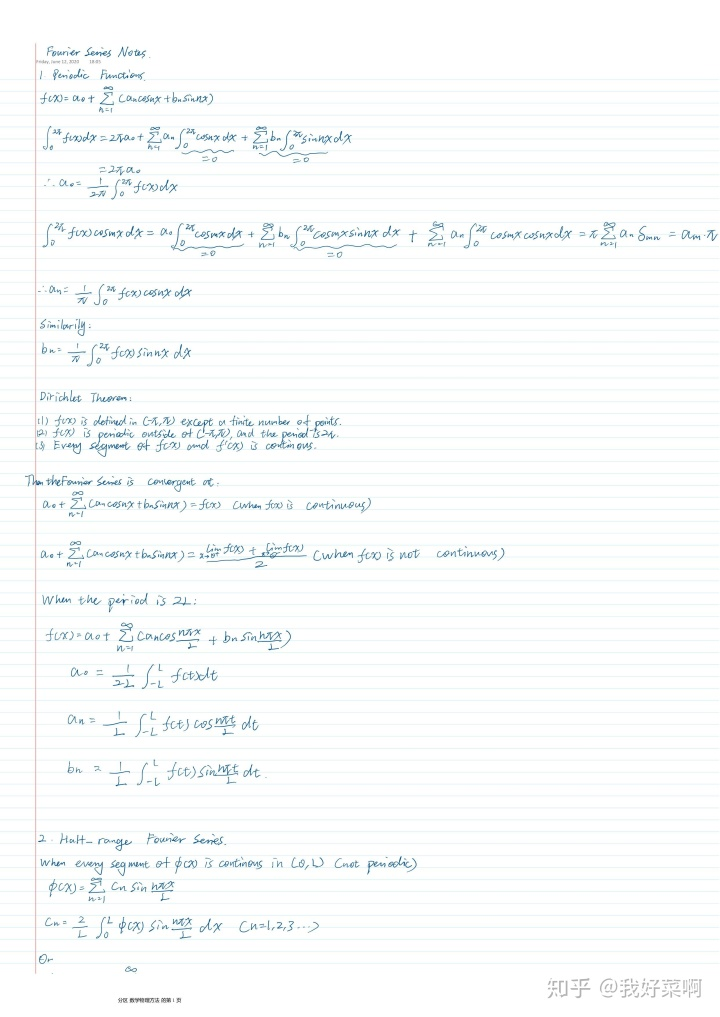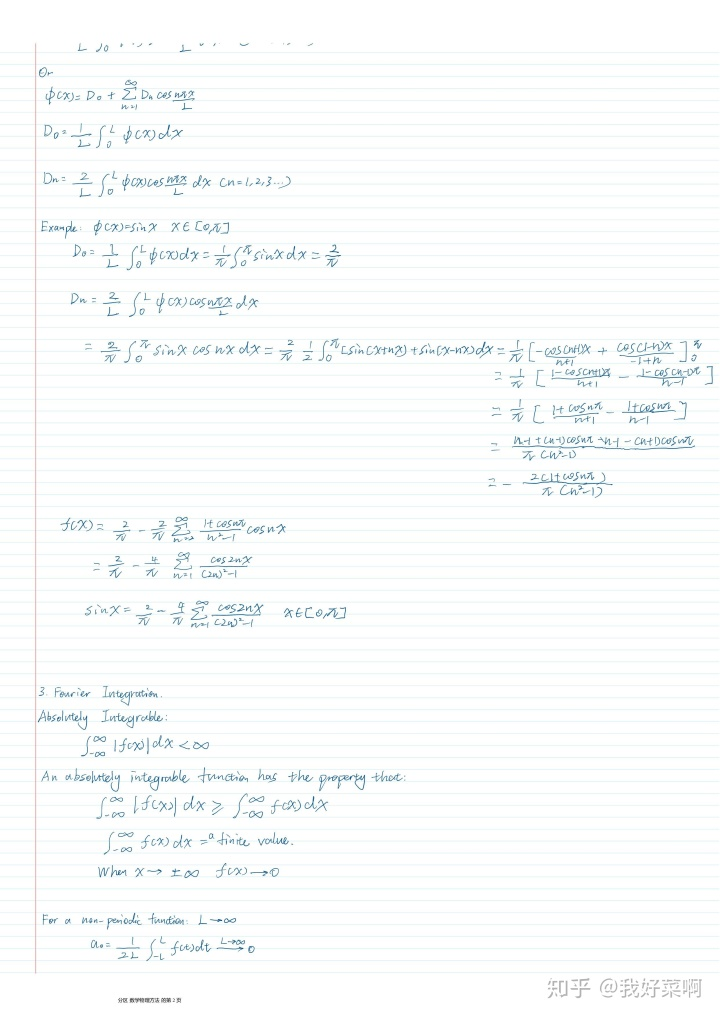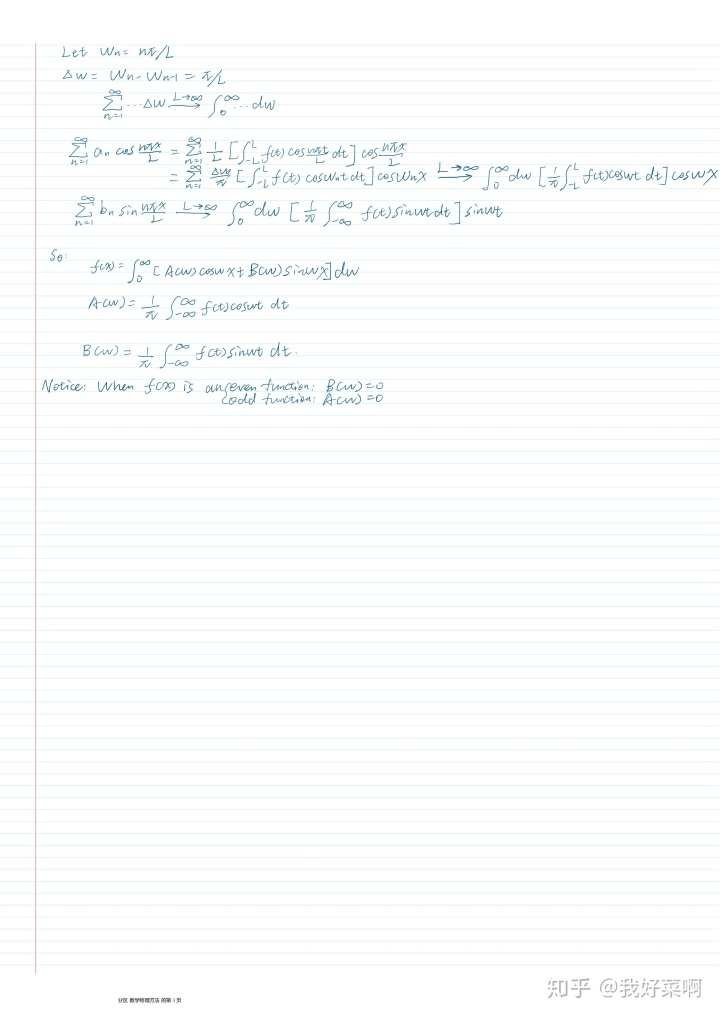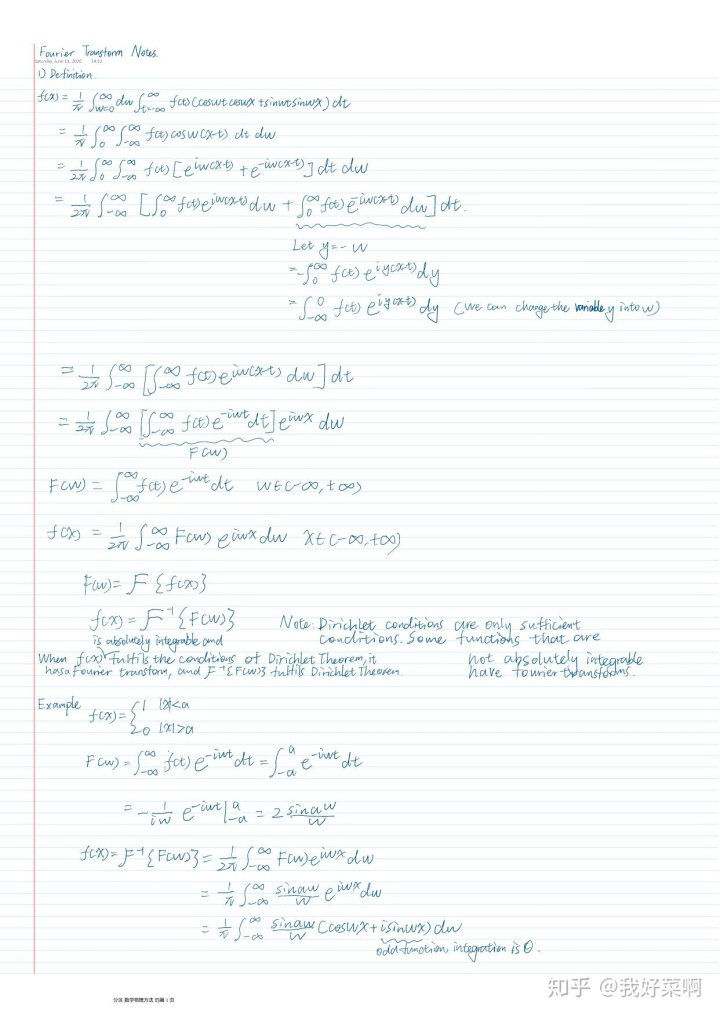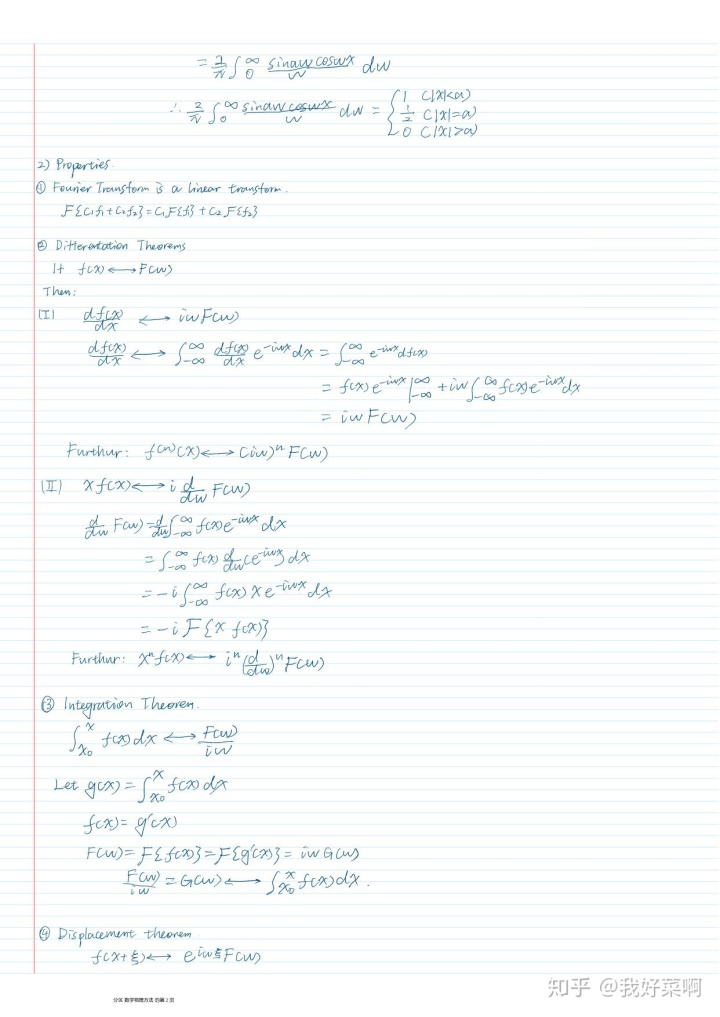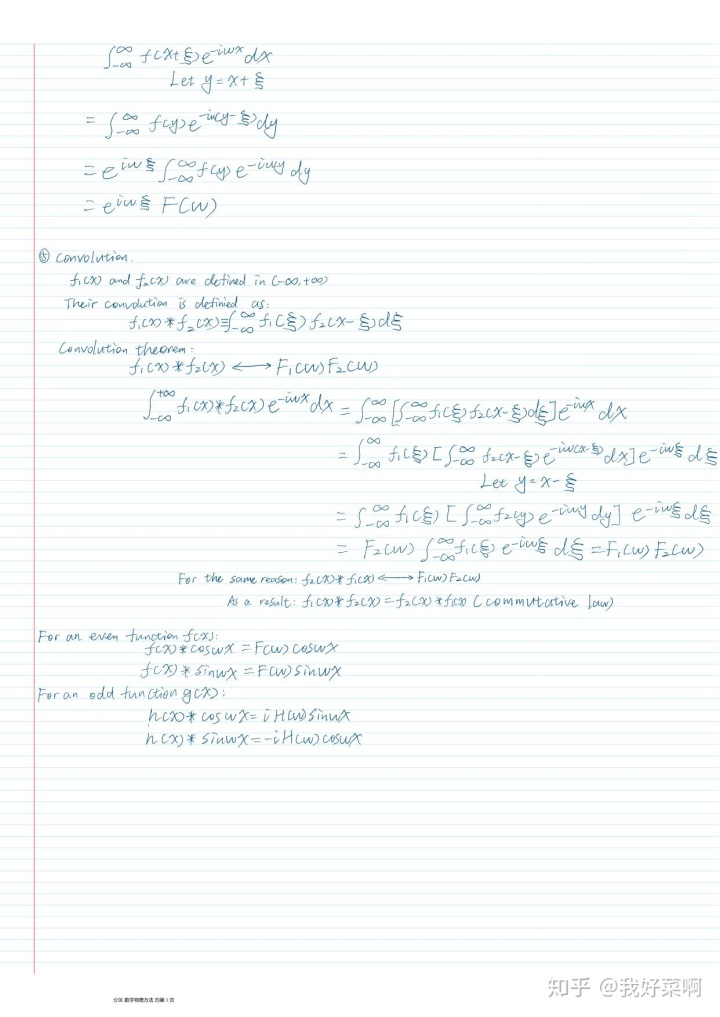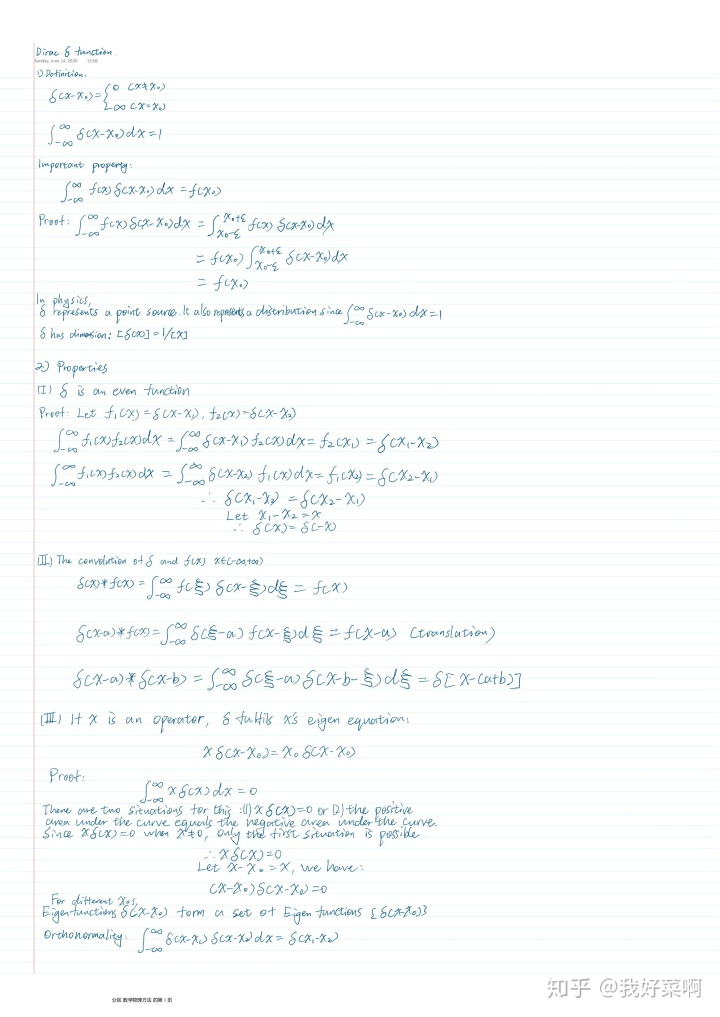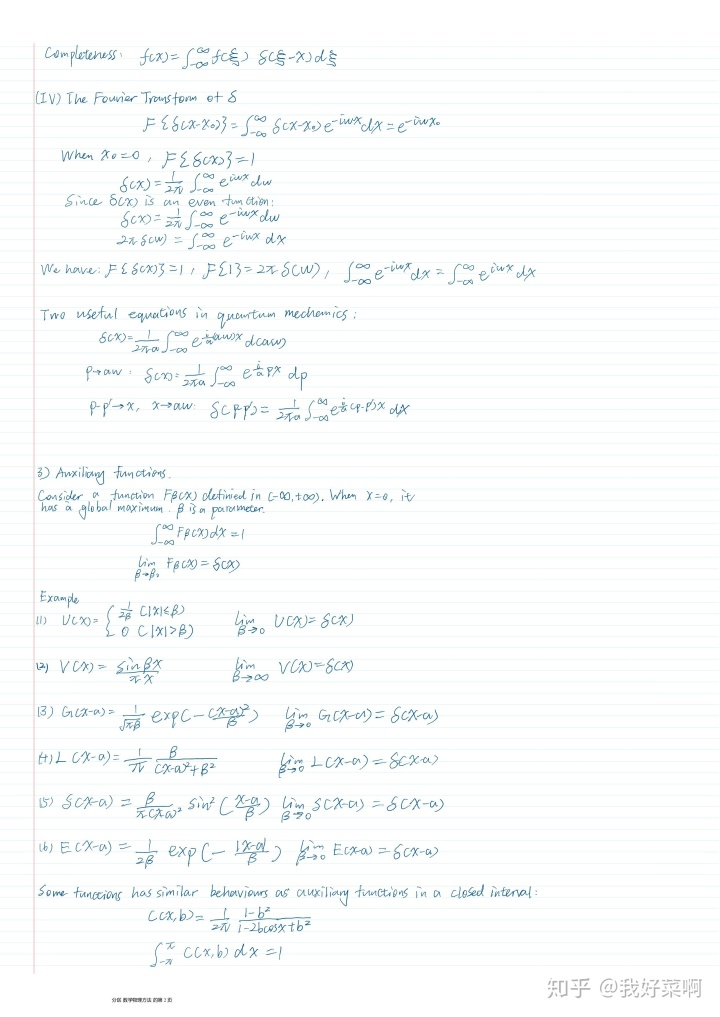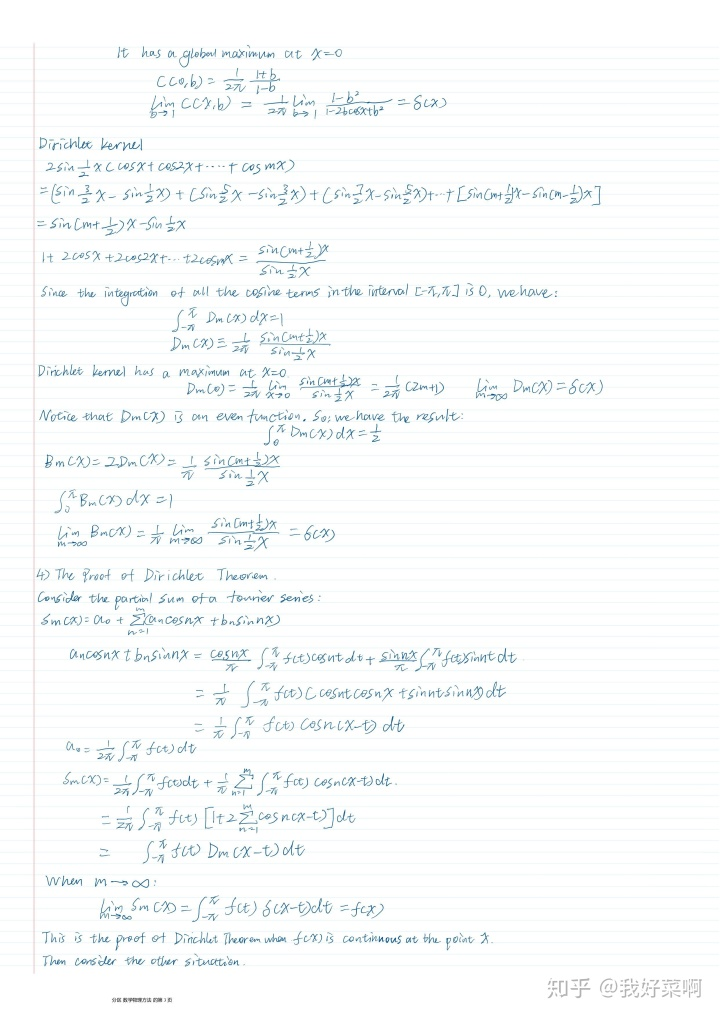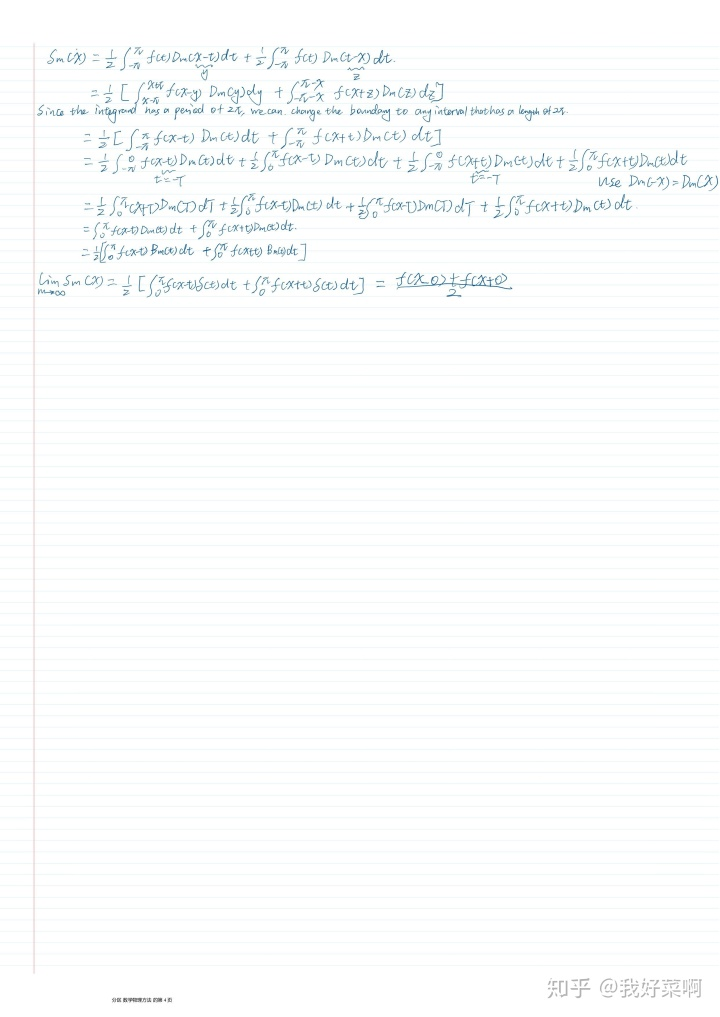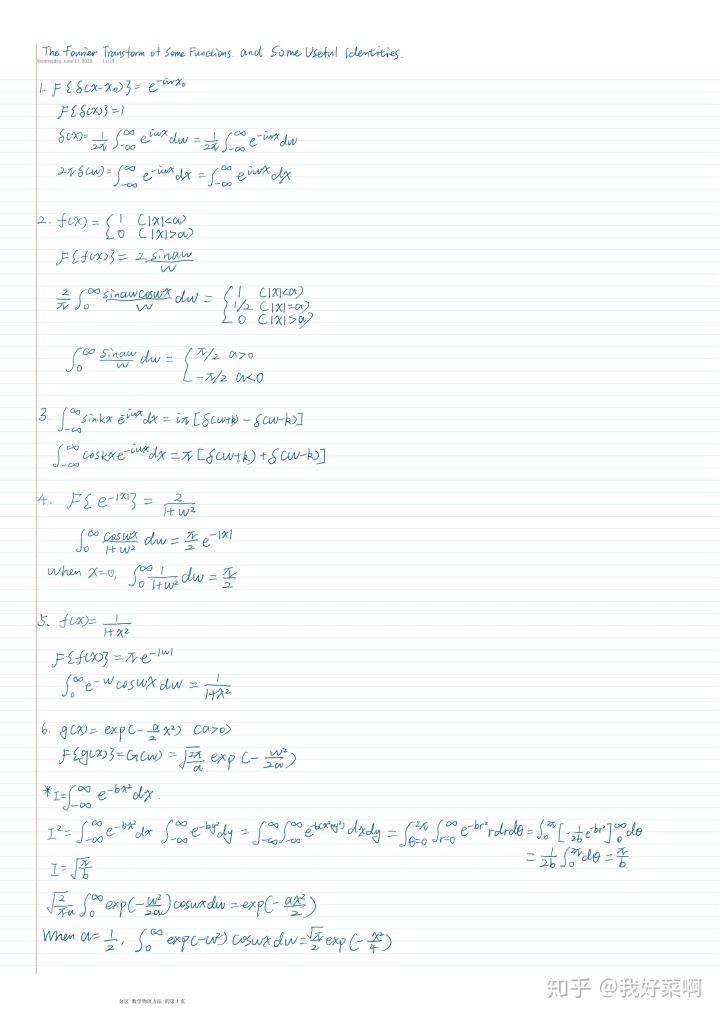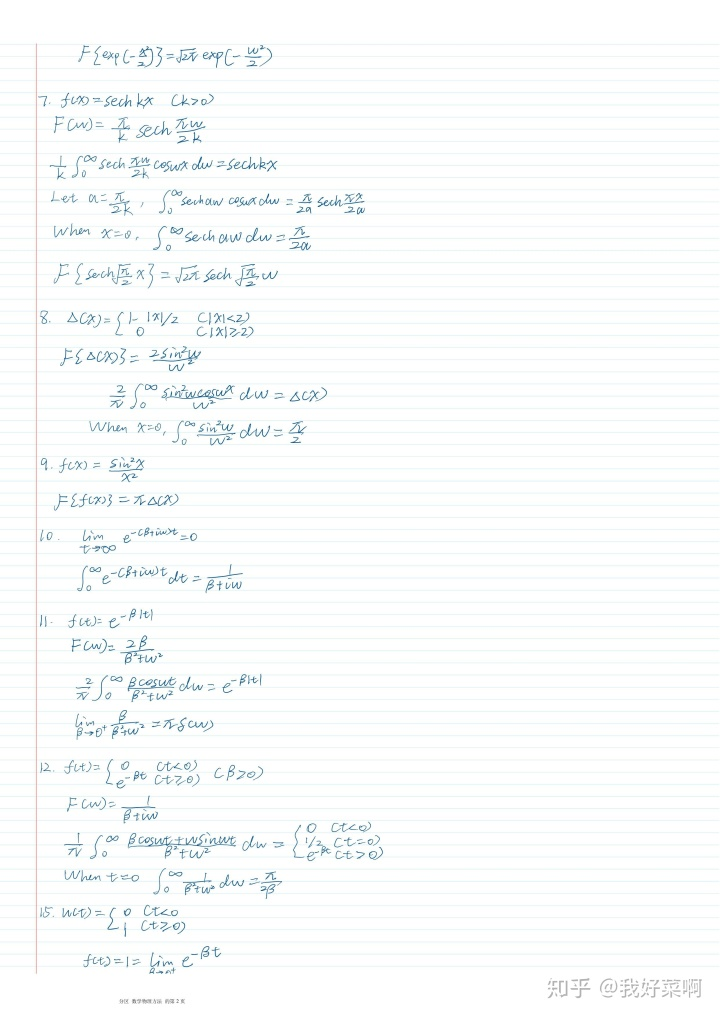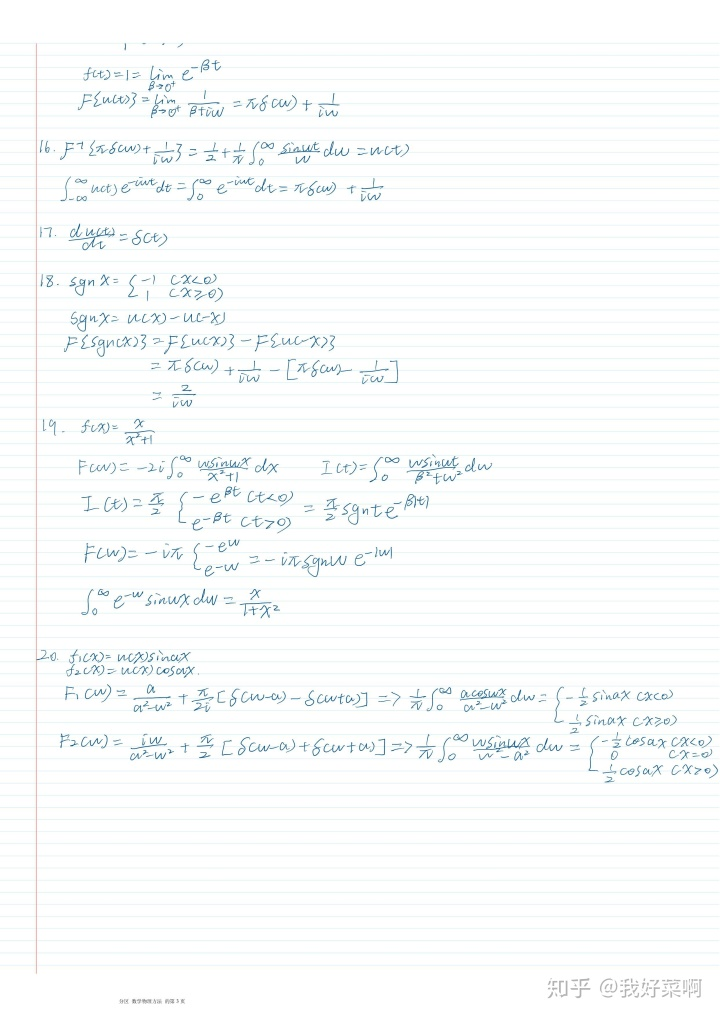笔记中没有包含傅里叶变换的应用。这里还是应该稍微提一下。第一，把频谱进行反变换可以得到原函数，由此可以证明很多积分公式（这些积分公式都包含在笔记中了，但是推导全都省略了）。第二，傅里叶变换可以被用来求解一些微分和积分方程。在方程两边同时取傅里叶变换，由于傅里叶变换的导数和积分性质，取了傅里叶变换以后的方程就变成了关于频谱的普通代数方程。直接计算出频谱以后，利用傅里叶反变换可以求出原函数，即方程的解。最后，感谢老妈给我从国内寄的书。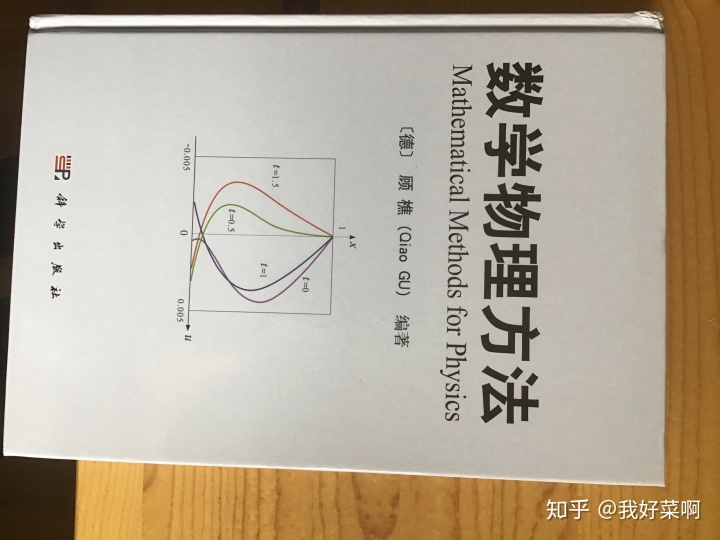展开全文• 文章目录深刻理解傅里叶级数与傅里叶变换的联系一、引入1.1 信号分解的基本思想1.2 系统特征函数1.3 复指数分解二、周期信号的傅里叶级数2.1 谐波复指数集2.2 傅里叶级数2.2.1 表示形式2.2.2 收敛条件2.2.3 傅里叶...
文章目录一、引入1.1 信号分解的基本思想1.2 系统特征函数1.3 复指数分解二、周期信号的傅里叶级数2.1 谐波复指数集2.2 傅里叶级数2.2.1 表示形式2.2.2 收敛条件2.2.3 傅里叶系数三、傅里叶变换3.1 周期矩形脉冲信号3.2 傅里叶变换对四、傅里叶级数与傅里叶变换的联系4.1 信号三参数4.2 几何直观
一、引入
1.1 信号分解的基本思想
信号分析的基本思想之一是将复杂信号用基本信号表示，这样就能通过简单信号的性质来分析复杂信号。这里要求基本信号应具有：
（1）由这些基本信号能够构成相当广泛的一类信号
（2）线性时不变系统(LTIS)对基本信号的响应应当十分简单，以使其对任意输入信号的响应都有很方便的表达式
举例而言，基本信号可以是冲激函数
$x\left( t \right) =\int{x\left( \tau \right)}\delta \left( t-\tau \right) d\tau$
上式即为连续时间信号的冲激分解，利用的是冲激函数的采样性质
将信号冲激分解后，就可以简单地利用冲激响应来表示输出：
$y\left( t \right) =\int{x\left( \tau \right)}h\left( t-\tau \right) d\tau$
1.2 系统特征函数
若系统对一个信号的响应仅为一个常数乘以该信号，则称该信号为此系统的特征函数，这个常数可以视作幅度因子，定义为特征值
1.3 复指数分解
按照1.1的思路，考察具有类似性质的基本信号——复指数
设激励$x\left( t \right) =e^{st}\,\, s\in C$，则输出通过上面说的冲激响应表示为：
$y\left( t \right) =\int{h\left( \tau \right)}x\left( t-\tau \right) d\tau =e^{st}\int{h\left( \tau \right) e^{-s\tau}}d\tau =e^{st}H\left( s \right)$
按照1.2的定义知道，复指数信号为LTIS的特征函数，对任一给定的$s$，常数$H(s)$为特征值；而对于一般的$s$，$H(s)$为关于$s$的函数，称为系统函数，当$s$为纯虚数时，$H(jw)$称为系统频率响应，下面要引入的傅里叶分析都是建立在$s=jw$的基础上，当$s$为一般复数时，考察的是拉普拉斯变换，本文不赘述。
那么复指数是否满足1.1节基本信号的要求呢？
为便于理解，先给出离散信号$x\left( t \right) =\sum_k{c_k}e^{s_kt}$，则响应$y\left( t \right) =\sum_k{c_kH\left( s_k \right)}e^{s_kt}$，即若信号可以进行复指数分解，则响应可表示为相同复指数的线性组合，系数与输入和频率响应相关
可见，复指数信号完美地符合了1.1的要求，在此基础上就建立起了傅里叶分析。
二、周期信号的傅里叶级数
2.1 谐波复指数集
设$x_0\left( t \right) =e^{j\omega _0t}$，定义基波频率为$\omega _0$，基波周期为$T_0$
令谐波信号集：
$\psi _k\left( t \right) =e^{jk\omega _0t}, k=0,\pm 1,\pm 2\cdots$
其中$k=0$时为直流分量，$k=\pm N$时为$N$次谐波分量
注意到谐波复指数集中，每一个信号都可以$T_0$为周期，这是因为：
$\psi _k\left( t \right) =e^{j\left( k\omega _0 \right) t}\\\Rightarrow T_k=\frac{2\pi}{|k|\omega _0}=\frac{T_0}{|k|}$
即每经过一个$T_0$，相当于经过了$|k|$个相应的谐波周期。因此，谐波复指数集的线性组合也就以$T_0$为周期：
$x\left( t \right) =\sum_{k=-\infty}^{+\infty}{a_k}e^{jk\omega _0t}\,\, \left( 1 \right)$
2.2 傅里叶级数
2.2.1 表示形式
在信号$x(t)$可以复指数分解的条件下研究此问题。考察(1)式，现实中绝大多数信号为实信号，因此认为$x(t)$为实数，满足：
$\overline{x\left( t \right) }=\overline{\sum_{k=-\infty}^{+\infty}{a_k}e^{jk\omega _0t}}$
由$x\left( t \right) =\overline{x\left( t \right) }$导出：
$x\left( t \right) =\sum_{k=-\infty}^{+\infty}{\overline{a_{-k}}e^{jk\omega _0t}}$
进一步：
$x\left( t \right) =a_0+\sum_{k=1}^{+\infty}{\left[ a_ke^{j\left( k\omega _0 \right) t}+\overline{a_ke^{j\left( k\omega _0 \right) t}} \right]}\\=a_0+2\sum_{k=1}^{+\infty}{\text{Re}\left\{ a_ke^{j\left( k\omega _0 \right) t} \right\}}$
(1)若$a_k$以极坐标形式给出，即$a_k=A_ke^{jw_0}$，此时
$x\left( t \right) =a_0+2\sum_{k=1}^{+\infty}{A_k\cos \left[ \left( k\omega _0 \right) t+\theta _k \right]}\,\,\,\left( 2 \right)$
(2)若$a_k$以笛卡尔坐标形式给出，即$a_k=B_k+jC_k$，此时
$x\left( t \right) =a_0+2\sum_{k=1}^{+\infty}{\left[ B_k\cos \left( k\omega _0t \right) -C_k\sin \left( k\omega _0t \right) \right]}\,\,\,\left( 3 \right)$
对于周期函数，(1)式即为傅里叶级数的复指数形式；(2)式为傅里叶级数的三角形式(极坐标下)；(3)式为傅里叶级数的三角形式(笛卡尔坐标下)。一般地，若信号能展开为傅里叶级数，其表示形式必为(1)(2)(3)之一
2.2.2 收敛条件
并非所有周期信号都可以级数展开，即，并非所有信号都可以进行复指数分解。一般而言，满足Dirchlet条件的信号必可进行傅里叶分析，不满足Dirchlet条件的信号没有傅里叶级数形式，但可能有傅里叶变换。
Dirchlet条件
(1)信号绝对可积
(2)在任何有限区间内，信号只有有限个最值
(3)在任何有限区间内，信号只有有限个不连续点，且每个不连续点处都只有有限值
2.2.3 傅里叶系数
若信号满足Dirchlet条件，必能复指数分解为：
$x\left( t \right) =\sum_{k=-\infty}^{+\infty}{a_k}e^{jk\omega _0t}$
现在问题在于傅里叶系数$a_k$的确定，可以采用以下方式求得：
$e^{-j\left( n\omega _0 \right) t}x\left( t \right) =\sum_{k=-\infty}^{+\infty}{a_k}e^{j\left( k-n \right) \omega _0t}\,\,$
两边同时在基波周期内积分：
$\int_T{e^{-j\left( n\omega _0 \right) t}x\left( t \right)}dt=\sum_{k=-\infty}^{+\infty}{\int_T{a_ke^{j\left( k-n \right) \omega _0t}dt}}\,\,\\\Rightarrow \int_T{e^{-j\left( n\omega _0 \right) t}x\left( t \right)}dt=\sum_{k=-\infty}^{+\infty}{a_k\int_T{\left[ \cos \left( k-n \right) w_0t+j\sin \left( k-n \right) w_0t \right] dt}}\,\,$
2.1节说过，谐波复指数集共同周期是基波周期，而三角函数一个周期内积分为0，在这里$T=|k-n|T_k$，因此等式左边在$k\ne n$时为0，$k=n$时为$T$，即：
$\int_T{e^{-j\left( n\omega _0 \right) t}x\left( t \right)}dt=a_nT$
由于$k=n$，所以改写为：
$a_k=\frac{1}{T}\int_T{e^{-j\left( k\omega _0 \right) t}x\left( t \right)}dt\,\, \left( 4 \right)$
此式即为傅里叶系数求解公式。
三、傅里叶变换
3.1 周期矩形脉冲信号图1
按照(4)式求解其傅里叶系数，得到：
$a_k=\frac{2E\sin \left( k\omega _0T_1 \right)}{k\omega _0T}$
从两个角度审视此式：
(1)视其为关于$k$的函数，即
$a(k)=\frac{2E\sin \left( k\omega _0T_1 \right)}{k\omega _0T}$
此时相当于将傅里叶系数等距离地排列在$k$轴上，因此当$T$趋于无穷时，$|a_k|$趋于0，即非周期信号的傅里叶系数幅度趋于0，正因如此，在幅度频谱中就看不出任何信息，所以对于非周期信号，不能仅关注$a_k$
(2)视其为包络线的采样图2
此时，视为：
$a_kT=\frac{2E\sin \left( \omega T_1 \right)}{\omega}\mid_{w=kw_0}^{}$
考虑关于$w$的函数$f\left( w \right) =\frac{2E\sin \left( \omega T_1 \right)}{\omega}$，$a_kT$就表示对$f(w)$上$w=kw_0$的位置进行采样。显然上面的采样间隔为$w_0=2\pi/T$，因此随着$T$不断增大，就出现了图2(a)->©取样变密的现象
重点理解的地方来了！！
注意这里$T$趋于无穷时，$|a_k|$依然趋于0，但可见的是$|a_k|T$是有限值(落在$f(w)$上)，因此$|a_k|T$的意义就是在$|a_k|$趋于0的情况下，通过T的加权作用，在一个有限的范围内显示出$|a_k|$间的相对大小关系，简言之，$|a_k|T$把肉眼不可见的非周期信号的傅里叶系数放大到肉眼可见，这其实就是傅里叶变换的引入基础。
3.2 傅里叶变换对
从3.1节知道，傅里叶变换的出发点，就是傅里叶系数的幅度加权与包络采样，因此：
$X\left( w \right) \mid_{w=kw_0}^{}=a_kT$
从而，
$X\left( w \right) =\int\limits_{-\infty}^{+\infty}{x\left( t \right) e^{-jwt}}dt\,\, \left( 5 \right)$
代入$x\left( t \right) =\sum_{k=-\infty}^{+\infty}{a_k}e^{jk\omega _0t}$中即得：
$x\left( t \right) =\frac{1}{2\pi}\int\limits_{-\infty}^{+\infty}{X\left( w \right) e^{jwt}}dw\,\, \left( 6 \right)$
(5)(6)式合称为一对傅里叶变换对，(5)式称为傅里叶变换积分
四、傅里叶级数与傅里叶变换的联系
4.1 信号三参数
这里定义信号的三参数为幅度、初相、频率(或角频率)，在傅里叶分析中，只要确定组成信号的所有复指数信号的三参数，就可以完全表征。无论是傅里叶级数还是傅里叶变换，事实上都是在求一个包含三参数的表达式来表示一个信号。
在傅里叶级数展开中，傅里叶系数表示了在频率$w=kw_0$时复指数信号的幅度和相位；在傅里叶变换中，傅里叶积分$X(w)$表示了全频率复指数信号的三参数信息——可以认为是公式化的频谱
具体来说，列于下表：

幅度
相位
频率

$a_k$
绝对值
绝对值

$X(w)$
加权相对值
绝对值
绝对值

事实上，不应该以信号的周期与否来割裂傅里叶变换与傅里叶级数。换言之，周期信号与非周期信号都有相应的傅里叶变换和傅里叶系数，只不过周期信号的傅里叶变换为冲激函数的线性组合，非周期信号的傅里叶系数趋于0，但有相对大小。
4.2 几何直观图3 傅里叶变换图4 傅里叶级数
几何直观上，傅里叶变换是连续函数，因为其对象是全频率；傅里叶级数是离散的，因为其对象是采样的部分频率。
最后
若有错误欢迎指出，若有疑问欢迎探讨，若需转载请告知。


展开全文信号处理
• 傅里叶级数与傅里叶变换 傅里叶级数 定义：满足狄利赫里条件的周期函数，可以变换成以三角函数或指数函数为基对周期函数的无穷级数展开 意义：通过傅里叶级数可以分析各个频率分量和直流分量 x(t)=∑n=−∞∞Xne−jn...信号处理
• 最近对傅里叶级数有了些疑惑，原本学习的时候就是只会记公式，但不明白数学和物理意义，更不明白怎么用，现在发现傅里叶变换真的太有用了，毕竟是时域和频域的连接，是一组非常高级的优美的数学公式。所以复习的时候...
• 傅里叶级数： 任何一个周期性的函数f(t)，可以表示为一系列正余弦函数的和。正余弦函数是一组正交基。 傅里叶变换 不具有周期性的信号可以看作是周期为无穷大的信号。 ......
• 1.三角函数正交性 2.周期为2pi的函数进行三角函数展开 3.周期为2L的函数进行三角函数展开 4.使用欧拉公式，复指数形式将周期为T的函数展开 5.傅里叶变换与傅里叶变换 ...
• 0x01 三角函数系的正交性与傅里叶级数 我们首先看一下这个定理: 组成三角级数的函数系 0(sin0x),1(cos0x),sinx,cosx,sin2x,cos2x,…,sinnx,cosnx \begin{aligned} 0(\text{sin}0x),1(\text{cos}0x),\text{sin}x,\...信号处理
• 最近对傅里叶级数有了些疑惑，原本学习的时候就是只会记公式，但不明白数学和物理意义，更不明白怎么用，现在发现傅里叶变换真的太有用了，毕竟是时域和频域的连接，是一组非常高级的优美的数学公式。所以复习的时候...
• 首先，傅里叶分析是指把一个周期...傅里叶级数 三角函数系的正交性 三角函数系：{1,sinx,cosx,sin2x,cos2x,…,sinnx,cosnx,…}，它由无数个sinnx和cosnx组成，其中n=0,1,2,…。 正交性： ∫−ππsin⁡nxcos⁡mxdx=0，经验分享
• 周期函数转换成傅里叶级数？ 展开的傅里叶级数的每个参数是如何得到的？ 三角函数的正交性
• 傅里叶分析可分为傅里叶级数（Fourier Serie）和傅里叶变换(Fourier Transformation) 傅里叶级数 傅里叶告诉我们，任何周期函数，都可以看作是不同振幅，不同相位正弦波的叠加。 最前面黑色的线就是所有正弦波叠加...python
• 知乎：https://www.zhihu.com/question/21665935
• 我们在量子力学的道路上已经越走越远了，但是在这一段时间的学习中我发现数学基础的扎实与否对我们进一步学习量子力学的相关知识起到了决定性的作用（似乎...，而是静下心来好好的研究一些数学知识——傅里叶分析...量子力学
• 在读本文前，请先大致浏览一下笔记篇里的东西，下面使用的符号及其意义都跟笔记篇里是一致的。笔记篇里记录的大都是基础的公式，教科书上都可以找到。   （抱歉，刚发现有点小错误：... 本文中提到的傅里叶级数都是
• 傅里叶级数的三角函数形式，傅里叶级数的复指数形式，
• 傅里叶级数与傅里叶变换_Part4_傅里叶级数的复数形式贴图如下 傅里叶级数与傅里叶变换_Part4_傅里叶级数的复数形式. 贴图如下经验分享...